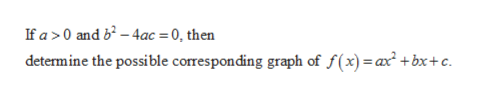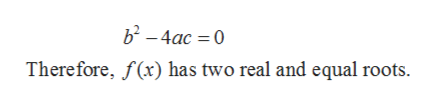If a>0 and b2-4ac=0, determine the possible corresponding graph of f(x)=ax2+bx+c.

Question

If a>0 and b2-4ac=0, determine the possible corresponding graph of f(x)=ax2+bx+c.

Step 1

Given:help_outlineImage TranscriptioncloseIf a >0 and b-4ac = 0, then determine the possible corresponding graph of f(x) a bx+c. fullscreen
Step 2

From the given information:help_outlineImage Transcriptioncloseb2-4ac 0 Therefore, f(x) has two real and equal roots. fullscreen
Step 3

Since, given polynomial is a quadratic polynomial therefore, the shape of graph of f(x) will be a parabola. Also given a > 0 hence, it will be open on the positive side of y-axis. Moreover, &...

Want to see the full answer?

See Solution

Want to see this answer and more?

Our solutions are written by experts, many with advanced degrees, and available 24/7

See Solution
Tagged in

Other